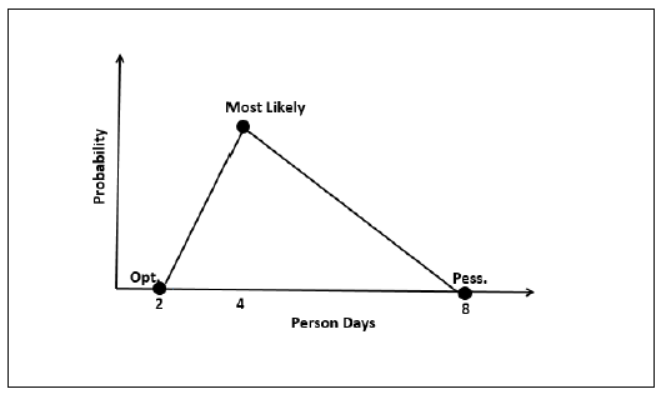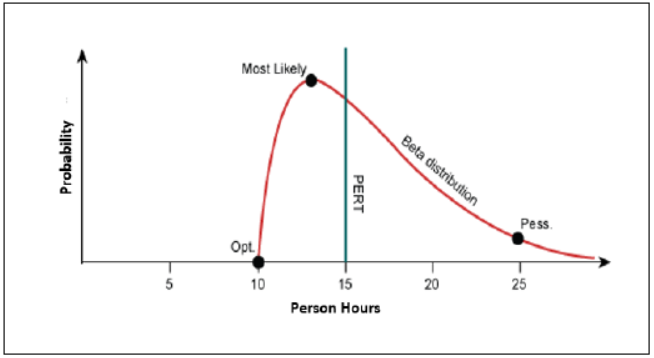{{announcement.body}}
{{announcement.title}}

# Estimation Techniques

DZone 's Guide to

# Estimation Techniques

### Give me a ballpark figure. Estimation is an important aspect of Agile development. Read about three data-driven methods this developer shares.

· Agile Zone ·
Free Resource

Comment (0)

Save
{{ articles.views | formatCount}} Views

Hello everyone. Recently, I was preparing for my PMP certification (which is still going on since last one year and still all modules are not complete) and I came across Time and Schedule Management module, which has one very useful topic of Estimation. The PMBOOK Guide says that we plan for Resource and Activity(s) and to perform Activity(s) we need to estimate the same.

Estimation is the process of finding an estimate, or approximation, which is a value that can be used for some purpose even if input data may be incomplete, uncertain, or unstable.

As per the definition of PMBOOK Guide:

" Estimation is the process of estimating the number of work periods needed to complete individual activities with estimated resources"

As per the PMBOOK Guide they are basically divided into 3 types:

1. Analogous Estimating
2. Parametric Estimation
3. Three Point Estimation

I'll take them one by one now.

## Analogous Estimation

This uses a similar past project information to estimate the duration or cost of your current project, hence the word, "analogy." You can use analogous estimation when there is limited information regarding your current project. Analogous estimation is a technique which uses the values of parameters from historical data as the basis for estimating similar parameters for a future activity. Parameters examples: Scope, cost, and duration. Measures of scale examples include size, weight, and complexity.

## Parametric Estimation

Parametric estimation is basically a type of estimation technique where we develop techniques/algorithms to evaluate different activities. This type of estimation is not having any standard way to estimate each project. In short, this estimation technique will differ from project to project and company to company. Key factors from which technique/algorithm is developed are:

• Quality of Data
• Sophistication of model

Function point and Delphi Technique are one of the type of Parametric Estimation.

### Function Point

A Function Point (FP) is a unit of measurement to express the amount of business functionality, an information system (as a product) provides to a user. FPs measure software size. They are widely accepted as an industry standard for functional sizing.

Function Point Analysis (FPA) technique quantifies the functions contained within software in terms that are meaningful to the software users. FPs consider the number of functions being developed based on the requirements specification.

Function Points (FP) Counting is governed by a standard set of rules, processes, and guidelines as defined by the International Function Point Users Group (IFPUG). These are published in the Counting Practices Manual (CPM).

There are two types of functions:

1. Data Functions

2. Transaction Functions

Data functions have 2 types:

• ILF (Internal Logical Files): This is data which are totally in boundaries of application. These aredirect orderived data from application/project.
• ELF (ExtrenalLogical Files): These are those data which has to be taken from outside the scope of application. Ex. Any application is taking data from API, whereapplicationis only known about the contract but not aware of data which will be sent through that contract.

Transaction Functions are of 3 types:

• EI (External Inputs): Transaction Function in which Data goes “into” the application from outside the boundary to inside
• EO (External Outputs): Transaction function in which data comes “out” of the system
• EQ (External Inquiries): Transaction function with both input and output components that result in data retrieval

### RETs, DETs, FTRs

RETs: Record Element Type (RET) is the largest user identifiable subgroup of elements within an ILF or an EIF

DETs: Data Element Type (DET) is the data subgroup within an FTR. They are unique and user identifiable

FTRs: File Type Referenced (FTR) is the largest user identifiable subgroup within the EI, EO, or EQ that is referenced to.

### Elementary Process (EP)

Elementary Process is the smallest unit of functional user requirement that is meaningful to the user, contains at least one complete transaction.

All this together can be used to identify and calculate function points and its schedule. Explanation of whole method of calculation of Function Point will be in next post, as this is itself a one post full content to explain about this method.

### Delphi Method

Delphi Method is a structured communication technique, originally developed as a systematic, interactive forecasting method which relies on a panel of experts. The experts answer questionnaires in two or more rounds. After each round, a facilitator provides an anonymous summary of the experts’ forecasts from the previous round with the reasons for their judgments. Experts are then encouraged to revise their earlier answers in light of the replies of other members of the panel.

It is believed that during this process the range of answers will decrease and the group will converge towards the "correct" answer. Finally, the process is stopped after a predefined stop criterion (e.g. number of rounds, achievement of consensus, and stability of results) and the mean or median scores of the final rounds determine the results.

## Three-Point Estimation

Three-Point Estimation technique revolves around 3 parameters and they are:

1. Optimistic Outcome (tO): Outcome in the most ideal situation
2. Pessimistic Outcome (tP): Outcome in the worst case scenario
3. Most Likely Outcome (tM): Outcome in the most likely scenario.

TPE is basically divided into 2 types:

1. Triangular Technique (generally known as Three Point Estimation)
2. PERT (Program Evaluation & Review Technique)

### Triangular Technique

Based on the simple average and follows a triangular distribution.

E = (tO + tM + tP) / 3In Triangular Distribution:

• Mean = (tO + tM + tP) / 3

• Standard Deviation = √ [((tO − E)2 + (tM − E)2 + (tP − E)2) / 2]

• Mean and Standard Deviation has to be done on all activities present in WBS. To calculate Project level Estimation

• E (Project) = ∑ E (Task) and Project Level Standard Deviation as SD (Project) = √ (∑SD (Task)2)

### PERT (Program Evaluation & Review Technique)Weights most likely scenario 4 times more than an optimistic and pessimistic outcome.

• E = (tO + 4 × tM + tP)/6

• Standard Deviation (SD) measures the variability or uncertainty in the estimate.

• Mean = (tO + 4 × tM + tP)/6

• Standard Deviation (SD) = (tP − tO)/6

There steps to perform estimation which can be learned as there is no specific or standard way to divide steps for performing the Estimation Technique.

Topics:
agile, estimating and planning, estimation techniques, project management

Comment (0)

Save
{{ articles.views | formatCount}} Views

Opinions expressed by DZone contributors are their own.